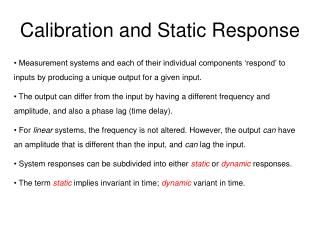# Calibration and Static Response - PowerPoint PPT PresentationDownload PresentationCalibration and Static Response

Calibration and Static ResponseDownload Presentation## Calibration and Static Response

- - - - - - - - - - - - - - - - - - - - - - - - - - - E N D - - - - - - - - - - - - - - - - - - - - - - - - - - -
##### Presentation Transcript

1. Calibration and Static Response • Measurement systems and each of their individual components ‘respond’ to inputs by producing a unique output for a given input. • The output can differ from the input by having a different frequency and amplitude, and also a phase lag (time delay). • For linear systems, the frequency is not altered. However, the output can have an amplitude that is different than the input, and can lag the input. • System responses can be subdivided into either static or dynamic responses. • The term static implies invariant in time; dynamic variant in time.

2. Figure 5.1 A Static Calibration • The slope of the static calibration curve is the staticsensitivity, K. • K=K(x) → many different values of K for a given curve. • Usually a high static sensitivity is desired (this leads to a lower x-from-y uncertainty).

3. Calibration Terms • Calibration is the comparison of a measured value to a known, • much more accurate value, which is called the true value. • The absolute error is the difference between the measured and true values. • The relative error is the ratio of the absolute error over the true value. • The accuracy of the calibration equals 1 - relative error. • When a calibration consists of a number of comparisons, the maximum • relative error often is used to characterize the calibration.

4. In-Class Example

5. Dynamic Response Characterization • The output, y(t), of a linear system can be determined by • by solving the linear ordinary differential equation (ODE) • in which the a’s are constant coefficients, n the order of the • system, and F(t) the forcing function. • y(t) can represent a physical variable that varies in time, • such as pressure, temperature, velocity, … • The exact ODE that describes the system is derived from • a conservation law.

6. This is known as Fourier’s law. Example First-Order System • Consider the response of a bulb thermometer • that is immersed from room air into hot water. • Heat is transferred from the hotter water through • glass wall into the cooler liquid inside the bulb. • This thermal energy transfer is governed by the first law of thermodynamics. E = total energy (J); Q = heat transfer (J); t = time (s) • Heat is transferred convectively to the glass from the hot water as h = heat transfer coefficient (W / [m2K]); A = surface area of heat transfer

7. Assume that all of the heat transferred from the hot water through the glass • reaches the liquid inside the bulb, thereby increasing the temperature of the • liquid. Conservation of energy requires that m = mass of the liquid (kg); Cv = specific heat at constant volume of the liquid (J / [kg K]) • Combining the previous equations gives • This is a linear, first-order ordinary differential equation, which is of the form Here, y = T, K = 1, Thw = F(t), and t = mCv/hA.

8. For a zero-order system (n=0), Examples: strain gage, pitot-static tube • For a first-order system (n=1), Examples: thermometer, RC circuit • For a second-order system (n=2), Examples: pressure transducer, accelerometer, RLC circuit Lower-Order Systems